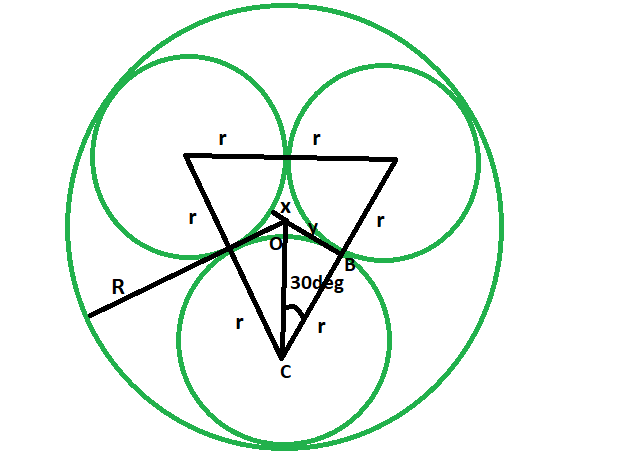GeeksforGeeks App
Open AppBrowser
Continue

# Radii of the three tangent circles of equal radius which are inscribed within a circle of given radius

Given here is a circle of a given radius. Inside it, three tangent circles of equal radius are inscribed. The task is to find the radii of these tangent circles.
Examples:

```Input: R = 4
Output: 1.858

Input: R = 11
Output:: 5.1095```Approach

• Let the radii of the tangent circles be r, and the radius of the circumscribing circle
is R.x is the smaller distance from the circumference of the tangent circle and the center of the circumscribing circle.
• From the diagram, it is very clear,
2r + x = R
• now in triangle OBC
cos 30 = r/(r+x)
rcos30 + xcos30 = r
x = r(1-cos30)/cos30
• also, x = R-2r
• So,
R-2r = r(1-cos30)/cos30
R-2r = 0.133r/0.867
R-2r = 0.153r
R = 2.153r
so, r = 0.4645R## C++

 `// C++ program to find the radii``// of the three tangent circles``// of equal radius when the radius``// of the circumscribed circle is given` `#include ``using` `namespace` `std;` `void` `threetangcircle(``int` `R)``{``    ``cout << ``"The radii of the tangent circles is "``         ``<< 0.4645 * R << endl;``}` `// Driver code``int` `main()``{``    ``int` `R = 4;``    ``threetangcircle(R);``    ``return` `0;``}`

Java

``````
// Java program to find the radii
// of the three tangent circles
// of the circumscribed circle is given
import java.io.*;

class GFG
{

static void threetangcircle(int R)
{
System.out.print("The radii of the tangent circles is "
+ 0.4645 * R);
}

// Driver code
public static void main (String[] args)
{
int R = 4;
threetangcircle(R);
}
}

// This code is contributed by anuj_67..

``````

## Python3

 `# Python3 program to find the radii``# of the three tangent circles``# of equal radius when the radius``# of the circumscribed circle is given` `def` `threetangcircle(R):``    ``print``(``"The radii of the tangent"``,``          ``"circles is "``, end ``=` `"");``    ``print``(``0.4645` `*` `R);` `# Driver code``R ``=` `4``;``threetangcircle(R);` `# This code is contributed``# by Princi Singh`

## C#

 `// C# program to find the radii``// of the three tangent circles``// of equal radius when the radius``// of the circumscribed circle is given``using` `System;` `class` `GFG``{` `static` `void` `threetangcircle(``int` `R)``{``    ``Console.WriteLine(``"The radii of the tangent circles is "``        ``+ 0.4645 * R);``}` `// Driver code``public` `static` `void` `Main ()``{``    ``int` `R = 4;``    ``threetangcircle(R);``}``}` `// This code is contributed by anuj_67..`

PHP

``````
<?php
// PHP program to find the radii
// of the three tangent circles
// of the circumscribed circle is given

function threetangcircle(\$R)
{
echo "The radii of the tangent circles is ",
( 0.4645 * \$R );
}

// Driver code
\$R = 4;
threetangcircle(\$R);

// This code is contributed by ihritik
?>

``````

## Javascript

 ``

Output:

`The radii of the tangent circles is 1.858`

Time Complexity: O(1)

Auxiliary Space: O(1)

My Personal Notes arrow_drop_up